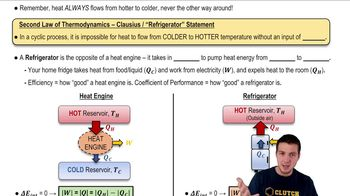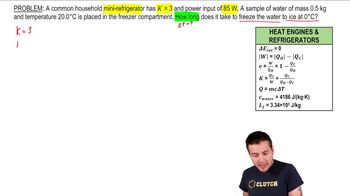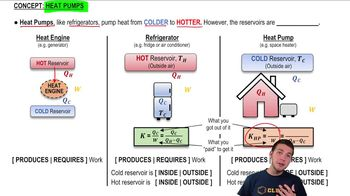Start typing, then use the up and down arrows to select an option from the list.
3:04 minutes
Problem 20d
Textbook Question

# The coefficient of performance K = H/P is a dimensionless quantity. Its value is independent of the units used for H and P, as long as the same units, such as watts, are used for both quantities. However, it is common practice to express H in Btu/h and P in watts. When these mixed units are used, the ratio H/P is called the energy efficiency ratio (EER). If a room air conditioner has K = 3.0, what is its EER?Verified Solution
This video solution was recommended by our tutors as helpful for the problem above.
36views

### Watch next

Master Refrigerators with a bite sized video explanation from Patrick Ford

Start learning06:4705:5506:15